# Foundations of Cryptography Lecture 14 Malleability Chosen Ciphertext

• Slides: 70Foundations of Cryptography Lecture 14: Malleability, Chosen Ciphertext Attacks, Cramer-Shoup Cryptosystem Lecturer: Moni Naor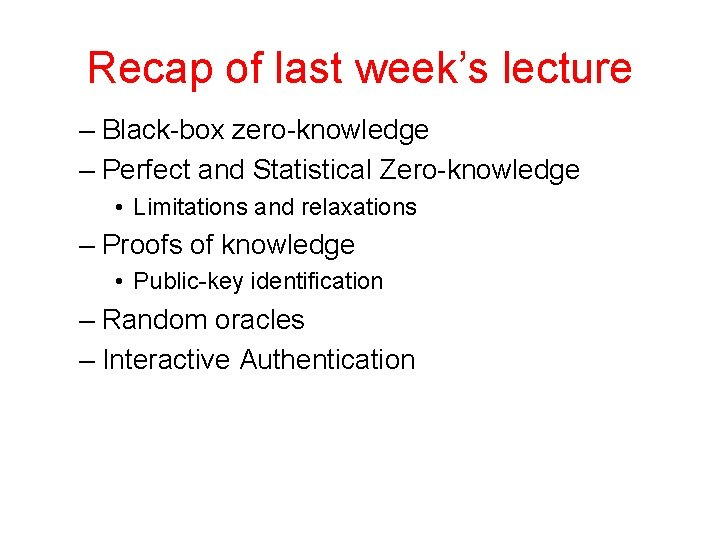Recap of last week’s lecture – Black-box zero-knowledge – Perfect and Statistical Zero-knowledge • Limitations and relaxations – Proofs of knowledge • Public-key identification – Random oracles – Interactive Authentication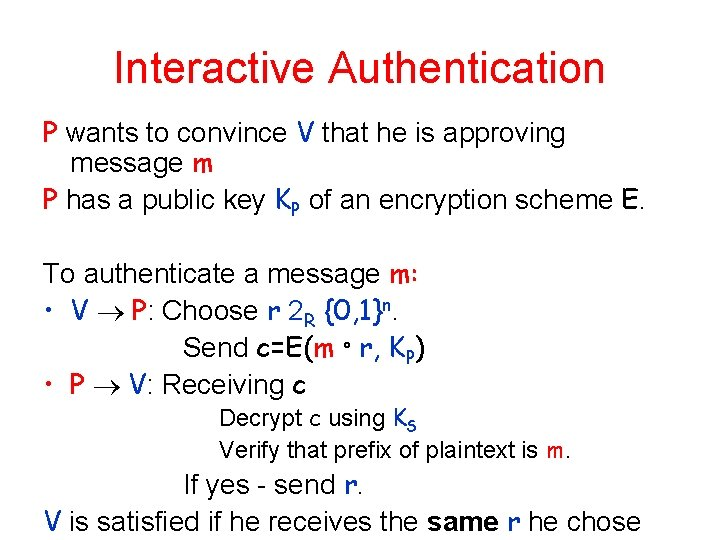Interactive Authentication P wants to convince V that he is approving message m P has a public key KP of an encryption scheme E. To authenticate a message m: • V P: Choose r 2 R {0, 1}n. Send c=E(m ° r, KP) • P V: Receiving c Decrypt c using KS Verify that prefix of plaintext is m. If yes - send r. V is satisfied if he receives the same r he chose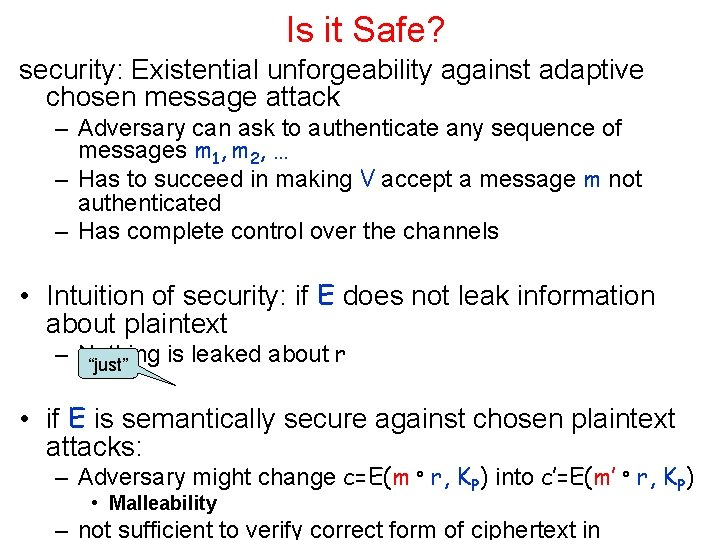Is it Safe? security: Existential unforgeability against adaptive chosen message attack – Adversary can ask to authenticate any sequence of messages m 1, m 2, … – Has to succeed in making V accept a message m not authenticated – Has complete control over the channels • Intuition of security: if E does not leak information about plaintext – Nothing is leaked about r “just” • if E is semantically secure against chosen plaintext attacks: – Adversary might change c=E(m ° r, KP) into c’=E(m’ ° r, KP) • Malleability – not sufficient to verify correct form of ciphertext inEncryption - Attacks • Chosen Plaintext – Minimal attack relevant to PKCs. Assumes decrypted messages remain secret. • CCA 1: Chosen Ciphertext - preprocessing mode (Lunch-break) – Challenge ciphertext is given after adversary relinquishes control of decryption device. – Good model for membership queries in computational learning. • CCA 2: Chosen Ciphertext - postprocessing mode – Challenge ciphertext is known when the attacks takes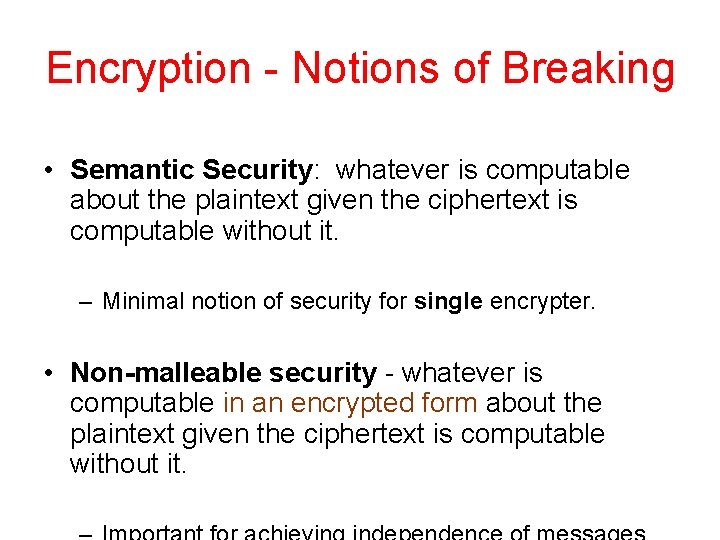Encryption - Notions of Breaking • Semantic Security: whatever is computable about the plaintext given the ciphertext is computable without it. – Minimal notion of security for single encrypter. • Non-malleable security - whatever is computable in an encrypted form about the plaintext given the ciphertext is computable without it.Application: auctions • Bidders submit their bids Highest one should independent win • Want to keep values secret until all bids submittedExample: Auctions Different requirements - different notions. • Semantic security is not sufficient for guaranteeing the independence of bids. • If key is used for a Single auction and secrecy is not required after the auction is over – – Non-malleable security against chosen plaintext attacks. • If key is used for many auctions and secrecy is not required after the auction is over – – Non-malleable security against – Chosen Ciphertext Attack in the preprocessing mode. • If key is used for many auctions and secrecy is required after the auction is over – Non-malleable security against – Chosen Ciphertext Attacks in the postprocessing mode.Semantic Security Whatever Adversary A can compute on encrypted string X 0, 1 n, so can A’ that does not see the encryption of X, A selects: • Distribution Dn on 0, 1 n • Relation R(X, Y) - computable in probabilistic polynomial time For every pptm A choosing a distribution Dn on 0, 1 n there is an pptm A’ so that for all pptm relation R for X R Dn Pr R(X, A(E(X)) - Pr R(X, A’( )) is negligible In other words: The outputs of A and A’ are indistinguishable even for a tester who is aware of XA: Dn A’: Dn E(X) X 2 R D n . A X A’ Y X R Y R ¼Non-Malleable Security Whatever Adversary A can compute on encrypted string X Encryp 0, 1 n, so can A’ that t does not see the encryption of X A selects: • Distribution Dn on 0, 1 n • Relation R(X, Y) - computable in probabilistic polynomial time For every pptm A choosing a distribution Dn on 0, 1 n there is an pptm A’ so that for all pptm relation R for X R Dn Pr R(X, D(A(E(X))) - Pr R(X, D(A’( ))) is negligible In other words: The outputs of A and A’ are indistinguishable even for a tester who gets the decryptions of what they output.A: Dn A’: Dn E(X) Deal with invalid ciphertext X X 2 R D n A A’ Y Y D R . X ¼ D RAll combinations are useful in some circumstances CPA Attack Breakin g Semanti c Combinations CCA 1 CCA 2 (lunch-time) (post-processing) Security Non. Malleabilit y All implications are proper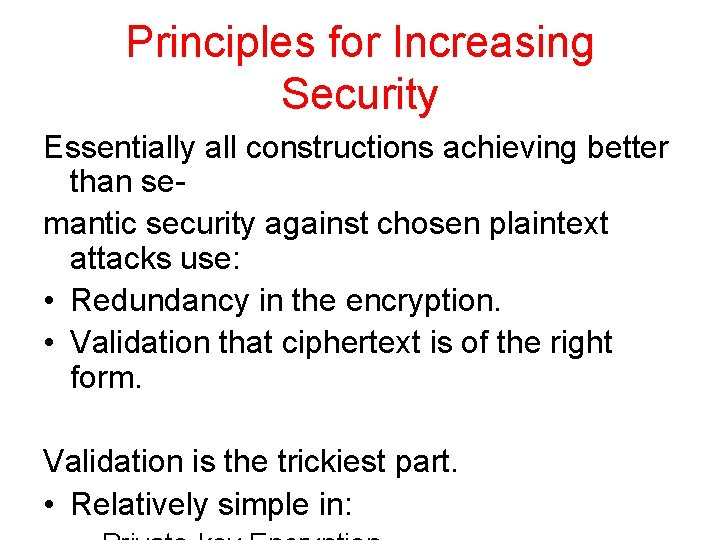Principles for Increasing Security Essentially all constructions achieving better than semantic security against chosen plaintext attacks use: • Redundancy in the encryption. • Validation that ciphertext is of the right form. Validation is the trickiest part. • Relatively simple in: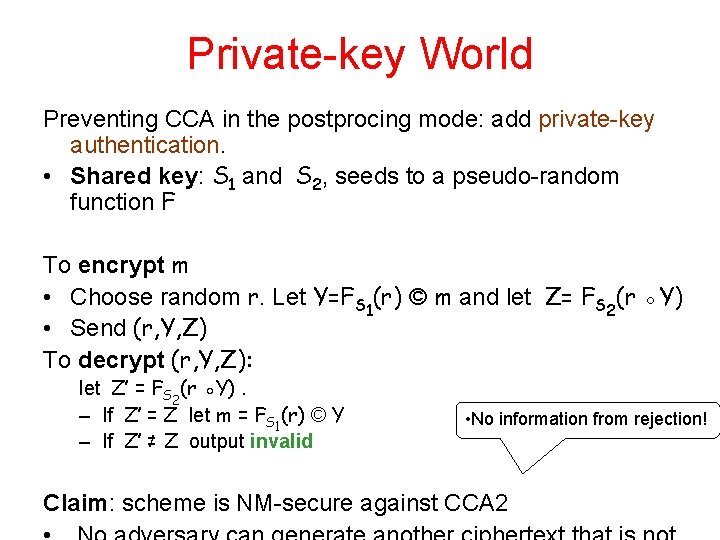Private-key World Preventing CCA in the postprocing mode: add private-key authentication. • Shared key: S 1 and S 2, seeds to a pseudo-random function F To encrypt m • Choose random r. Let Y=FS 1(r) © m and let Z= FS 2(r ◦Y) • Send (r, Y, Z) To decrypt (r, Y, Z): let Z’ = FS 2(r ◦Y). – If Z’ = Z let m = FS 1(r) © Y – If Z’ ≠ Z output invalid • No information from rejection! Claim: scheme is NM-secure against CCA 2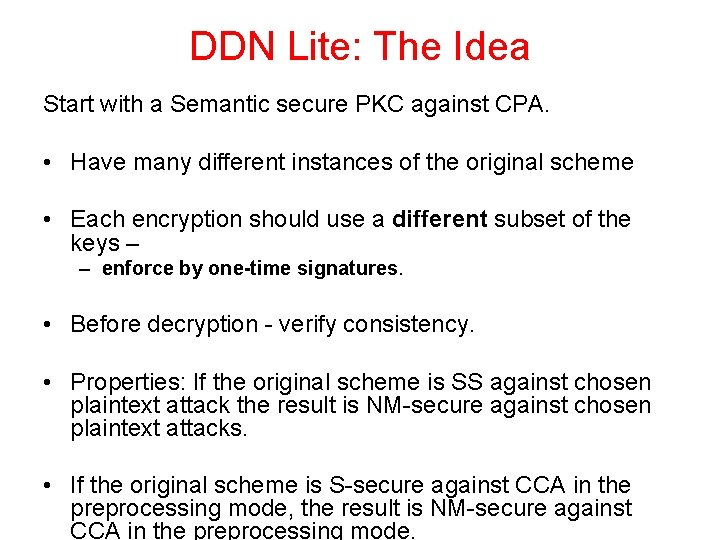DDN Lite: The Idea Start with a Semantic secure PKC against CPA. • Have many different instances of the original scheme • Each encryption should use a different subset of the keys – – enforce by one-time signatures. • Before decryption - verify consistency. • Properties: If the original scheme is SS against chosen plaintext attack the result is NM-secure against chosen plaintext attacks. • If the original scheme is S-secure against CCA in the preprocessing mode, the result is NM-secure against CCA in the preprocessing mode.DDN Lite Each is the public key of a SS PKC Public-Key: h. K 10, K 11 i, h. K 20, K 21 i, … h. Kn 0, Kn 1 i • A function h: {0, 1}* {0, 1}n - UOHWF Private-Key: Decryption keys of {Kib}. hard to find collision with target Encryption of a message m: input • Choose at random KOS, the public-key of a one-time signature scheme • Let b 1, b 2, … bn = h(K). • Encrypt m using keys Kibi to obtain C 1, C 2, …, Cn. • Sign h. C 1, C 2, … Cni using KOS-1 and h; Let S be the result • Ciphertext is: KOS, h. C 1, …, Cni, S. Decryption of ciphertext KOS, C 1, … Cn, S • Verify the signature S on h. C 1, C 2, … , Cni using KOS. • Verify the consistency (equality) of all the plaintexts. • Decrypt using any one of the keys.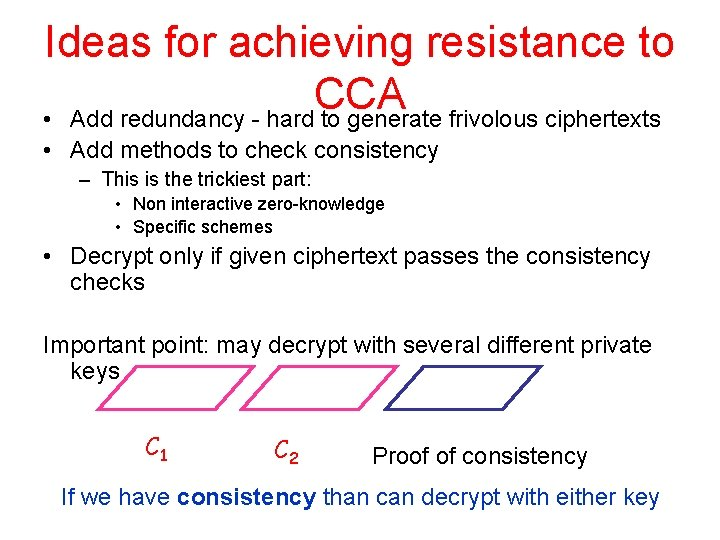Ideas for achieving resistance to CCA • Add redundancy - hard to generate frivolous ciphertexts • Add methods to check consistency – This is the trickiest part: • Non interactive zero-knowledge • Specific schemes • Decrypt only if given ciphertext passes the consistency checks Important point: may decrypt with several different private keys C 1 C 2 Proof of consistency If we have consistency than can decrypt with either keyProofs of consistency • How to have a proof of consistency that does not leak the plaintext – Non-Interactive Zero-Knowledge (NIZK) • How to make the proof itself nonmalleable – Ow can change it and get a different ciphertext with the same plaintext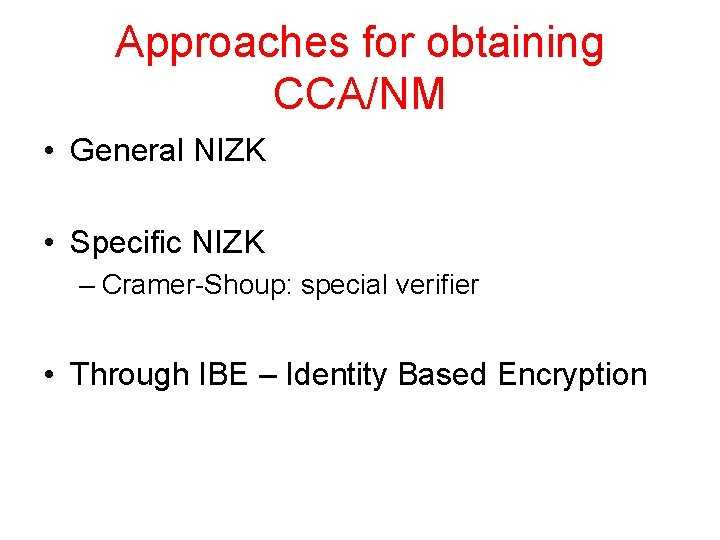Approaches for obtaining CCA/NM • General NIZK • Specific NIZK – Cramer-Shoup: special verifier • Through IBE – Identity Based EncryptionDiscrete Log Problem • Let G be a group and g an element in G. • Let y=gz and x the minimal non negative integer satisfying the equation. x is called the discrete log of y to base g. • Example: y=gx mod p in the multiplicative group of Zp • In general: easy to exponentiate via repeated squaring – Consider binary representation • What about discrete log? – If difficult, f(g, x) = (g, gx) is a one-way function DL Assumption for group G: • No efficient algorithm can solve for X [0. . n-1] whpDiscrete Log Problem Very useful group for DL: • P and Q: Large primes, s. t. Q | P-1 • g: an element of order Q in ZP*. Best known algorithms – Q or – subexponential in log P Randomized reduction: given Y generate Y’ = Ygr for r R [Q]Diffie-Hellman The Diffie-Hellman assumption Let G be a group and g an element in G. Given g, X=ga and Y=gb it is hard to find Z=gab for random a and b the probability of a poly-time machine outputting gab is negligible More accurately: a sequence of groups Don’t know how to verify whether given Z’ is equal to gabDecisional Diffie-Hellman Problem For for generator g of a group of size Q and a, b [Q] Given g, Y=ga, X=gb and Z decide whether Z =gab or Z gab Equivalent: is logg Y = log. X Z DDH-Assumption: • The DDH-Problem is hard in the worst case.Average DDH For a, b R [Q] and c which is either – c= ab – c R [Q] Given Y=ga and X=gb and Z =gc decide whether Z =gab or Z gab DDH-Assumption average case: • The DDH-Problem is hard for above distributionWorst to Average case reduction Theorem: The average case and worst case of the DDH-Assumption are equivalent. • Given ga and gb and gc (and P, Q) • Sample r, s 1, s 2 R [Q] c is either ab or not • compute a’ = ra s 1 mod Q ga’ = (ga)r gs 1 b’ = b s 2 mod Q a’b’=rab+ras 2+bs 1+s 1 s 2 b’ b s g = (g ) g 2 gc’ = (gc)r (ga)rs 2 (gb)s 1 gs 1 s 2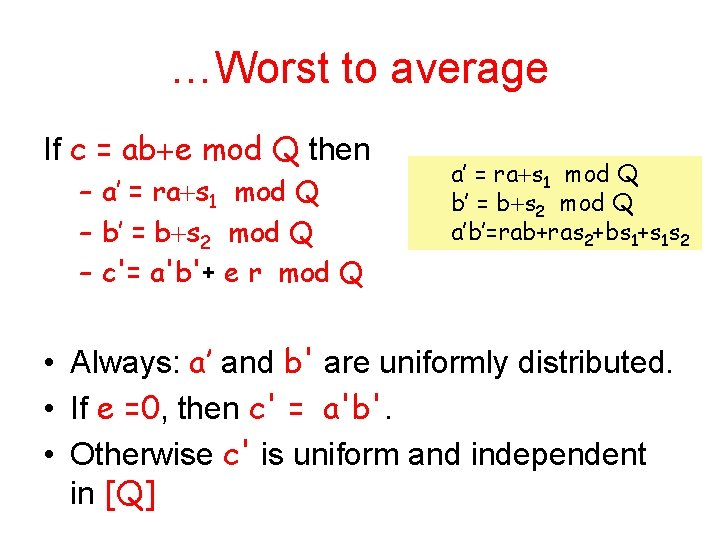…Worst to average If c = ab e mod Q then – a’ = ra s 1 mod Q – b’ = b s 2 mod Q – c'= a'b'+ e r mod Q a’ = ra s 1 mod Q b’ = b s 2 mod Q a’b’=rab+ras 2+bs 1+s 1 s 2 • Always: a’ and b' are uniformly distributed. • If e =0, then c' = a'b'. • Otherwise c' is uniform and independent in [Q]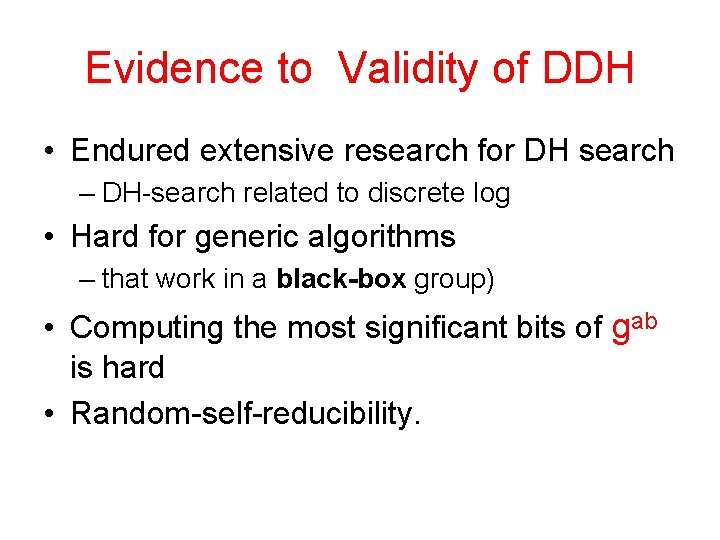Evidence to Validity of DDH • Endured extensive research for DH search – DH-search related to discrete log • Hard for generic algorithms – that work in a black-box group) • Computing the most significant bits of gab is hard • Random-self-reducibility.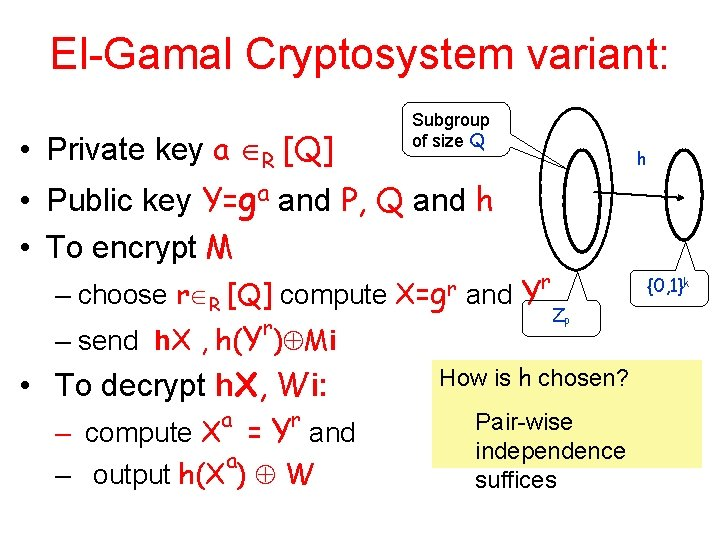El-Gamal Cryptosystem variant: • Private key a R [Q] Subgroup of size Q h • Public key Y=ga and P, Q and h • To encrypt M r – choose r R [Q] compute X=gr and Y Z r – send h. X , h(Y ) Mi How is h chosen? • To decrypt h. X, Wi: a r Pair-wise – compute X = Y and P a – output h(X ) W independence suffices {0, 1}k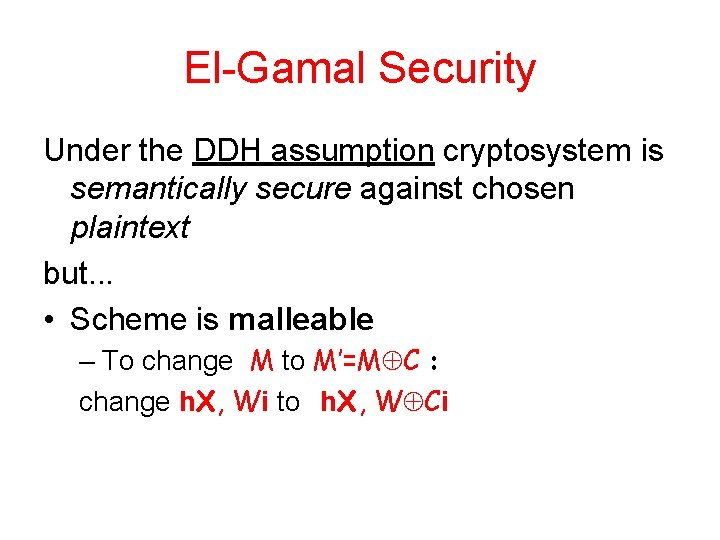El-Gamal Security Under the DDH assumption cryptosystem is semantically secure against chosen plaintext but. . . • Scheme is malleable – To change M to M’=M C : change h. X, Wi to h. X, W Cigenerators Proving consistency of exponentiations • Given g 1, g 2, X 1 , X 2 Is there is an r where X 1=g 1 r and X 2=g 2 r Honest verifier zero-knowledge proof: Verifier sends Z = g 1 b 1 g 2 b 2 for random b 1, b 2 Prover sends V = Zr Verifier accepts iff X 1 b 1 X 2 b 2 = V Leaks only a linear equation for b 1 and b 2 Simulator: choose random b 1, b 2 and output (g 1 b 1 g 2 b 2 , X 1 b 1 X 2 b 2 )Proving consistency of exponentiations • Given g 1, g 2, X 1 , X 2 Is there is an r where X 1=g 1 r and Z can be known when r and X are chosen! X 2=g 2 X 1 2 Honest verifier zero-knowledge proof: Verifier sends Z = g 1 b 1 g 2 b 2 for random b 1, b 2 Prover sends V = Zr Verifier accepts iff X 1 b 1 X 2 b 2 = V Leaks only a linear equation for b 1 and b 2 Soundness: if X 1=g 1 r and X 2=g 2 r+e then X 1 b 1 X 2 b 2 = g 1 rb 1 g 2(r+e)b 2 = g 1 rb 1 g 2 rb 2 g 2 eb 2 = Zr (g 2 e ) b 2 Random in the groupCramer-Shoup Lite • Private key: a, b 1, b 2 R [Q] • Public key: – g 1, g 2, Y=g 1 a and Z=g 1 b 1 g 2 b 2 • To encrypt M – choose r R [Q] r r – compute Y , X 1=g 1 r, X 2=g 2 r and Z r r h. X 1, X 2, h(Y ) M, Z i • To decrypt h. X 1, X 2, W, Vi – send – check validity: yes a X 1 b 1 X 2 b 2 = V and if r rCramer-Shoup Complexity • Encryption: 4 modular exponentiations • Decryption: 3 modular exponentiationsCramer Shoup Security Under the DDH assumption cryptosystem is semantically secure against chosen plaintext Show that the scheme secure against chosen ciphertext attacks (preprocessing) but. . . • Scheme is malleable – To change M to M’=M C change h. X, Wi to h. X, W CiChosen Ciphertext Attacks Lunchtime Adversary T has temporary access to decryption oracle. Then it is given a challenge • Semantic security - adversary chooses two message h. M 0, M 1 i • For d R 0, 1 it is given E(Md) and has to guess d. Let pd Pr T(E(Md )) ‘ 1’ d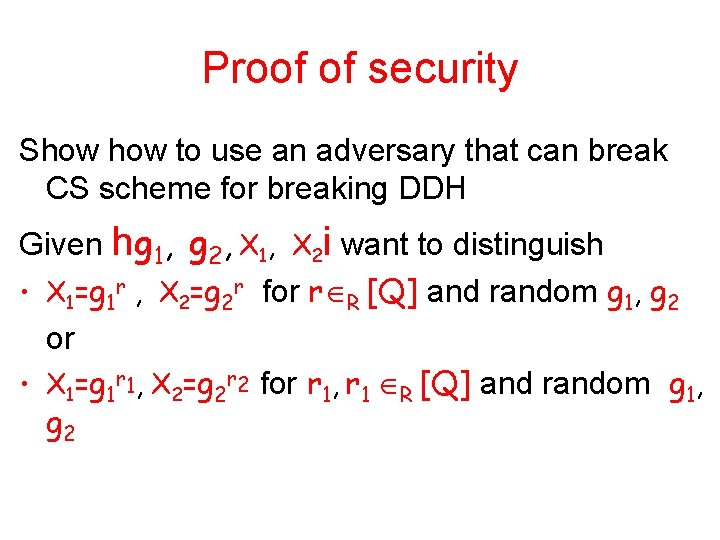Proof of security Show to use an adversary that can break CS scheme for breaking DDH Given hg 1, g 2, X 1, X 2 i want to distinguish • X 1=g 1 r , X 2=g 2 r for r R [Q] and random g 1, g 2 or • X 1=g 1 r 1, X 2=g 2 r 2 for r 1, r 1 R [Q] and random g 1, g 2. . . Proof of security - simulation Given hg 1, g 2, X 1, X 2 i generate • Private key a 1, a 2, b 1, b 2 R [Q] and • Public key hg 1, g 2, Y = g 1 a 1 g 2 a 2, Z=g 1 b 1 g 2 b 2 i • To decrypt h. X’ 1, X’ 2 , W, V i – check X’ 1 b 1 X’ 2 b 2 = V and if pass a a r r compute X’ 1 1 X’ 2 2 = Y. Output h(Y ) W Normal operation, independent of X 1, X 2. . . Proof of security - simulation When adversary chooses h M 0 , M 1 i : Generate For d R 0, 1 the ciphertext h X 1, X 2 , a h(X 1 1 a X 2 2 ) Md , V = b X 1 1 X 2 b 2 i Claim: If logg X 1 = logg X 2 then ciphertext is valid If logg X 1 logg X 2 then ciphertext is 1 2 inconsistent and independent of d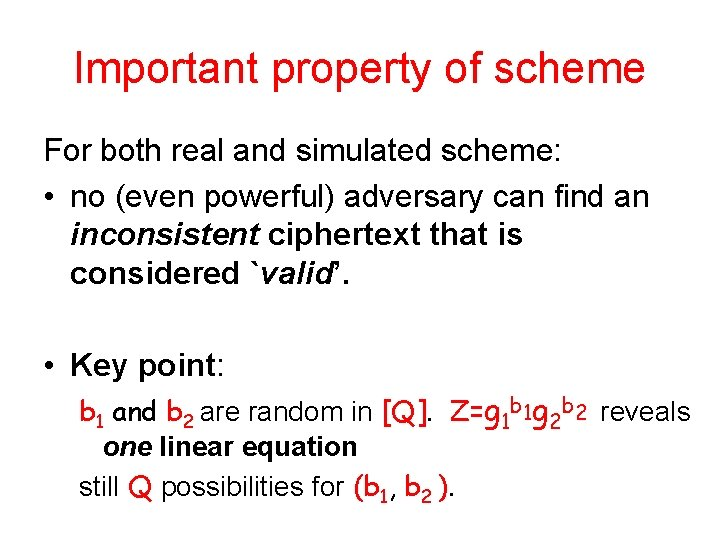Important property of scheme For both real and simulated scheme: • no (even powerful) adversary can find an inconsistent ciphertext that is considered `valid’. • Key point: b 1 and b 2 are random in [Q]. Z=g 1 b 1 g 2 b 2 reveals one linear equation still Q possibilities for (b 1, b 2 ).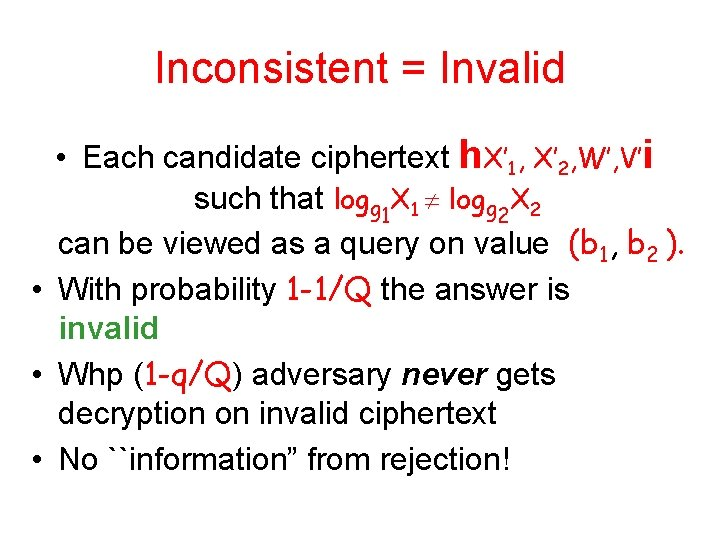Inconsistent = Invalid • Each candidate ciphertext h. X’ 1, X’ 2, W’, V’i such that logg 1 X 1 logg 2 X 2 can be viewed as a query on value (b 1, b 2 ). • With probability 1 -1/Q the answer is invalid • Whp (1 -q/Q) adversary never gets decryption on invalid ciphertext • No ``information” from rejection!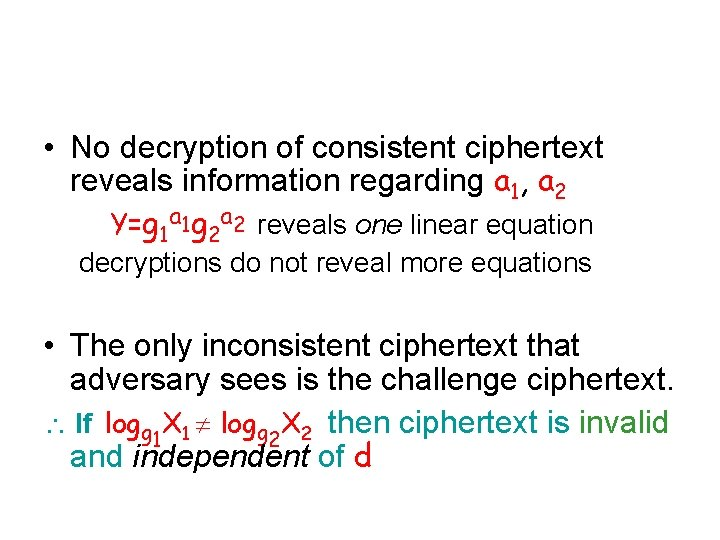• No decryption of consistent ciphertext reveals information regarding a 1, a 2 Y=g 1 a 1 g 2 a 2 reveals one linear equation decryptions do not reveal more equations • The only inconsistent ciphertext that adversary sees is the challenge ciphertext. If logg X 1 logg X 2 then ciphertext is invalid 1 2 and independent of d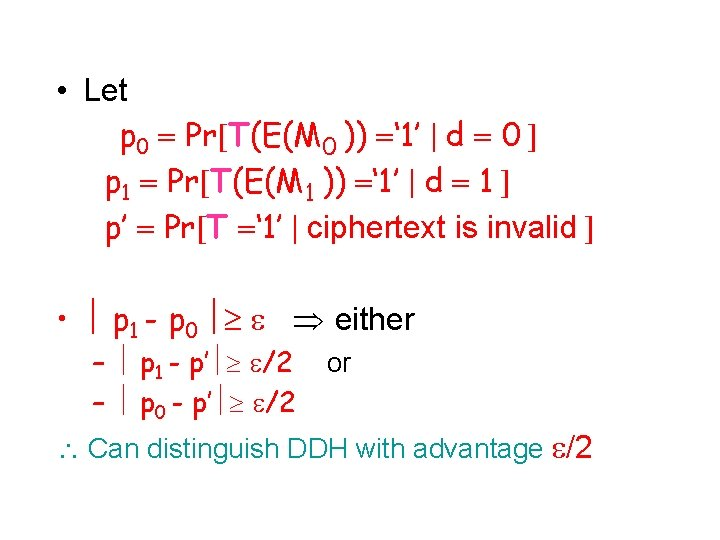• Let p 0 Pr T(E(M 0 )) ‘ 1’ d 0 p 1 Pr T(E(M 1 )) ‘ 1’ d 1 p’ Pr T ‘ 1’ ciphertext is invalid • p 1 - p 0 either – p 1 - p’ /2 – p 0 - p’ /2 or Can distinguish DDH with advantage /2Cramer-Shoup: Full Strength • Private key: a, b 1, b 2, c 1, c 2 R [Q] • Public key: – g 1, g 2, Y=g 1 a, Z=g 1 b 1 g 2 b 2, Z’=g 1 c 1 g 2 c 2 and H A UOWHF • To encrypt M, choose r R [Q] and r r – compute Y , X 1=g 1 X 2=g 2 W = h(Y ) M r, =H(W, X 1 , X 2 ) – send r, h. X 1, X 2 , W, Zr Z’ ri • To decrypt h. X 1, X 2, W, Vi – check validity: X 1 a b + c 1 1 r X 1 One-way hash b + c 2 2 r = V and if pass – compute X 1 = Y. Output h(Y ) WCramer Shoup (full strength) Security Under the DDH assumption cryptosystem is • non-malleable against • chosen ciphertext attacks in postprocessing mode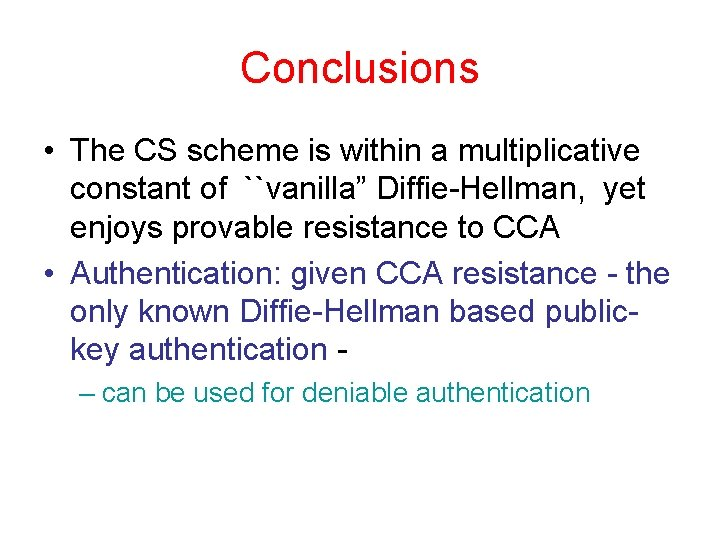Conclusions • The CS scheme is within a multiplicative constant of ``vanilla” Diffie-Hellman, yet enjoys provable resistance to CCA • Authentication: given CCA resistance - the only known Diffie-Hellman based publickey authentication – can be used for deniable authenticationInteractive Authentication P wants to convince V that he is approving message m P has a public key KP of an encryption scheme E. To authenticate a message m: • V P: Choose r 2 R {0, 1}n. Send c=E(m ° r, KP) • P V: Receiving c Decrypt c using KS Verify that prefix of plaintext is m. If yes - send r. V is satisfied if he receives the same r he choose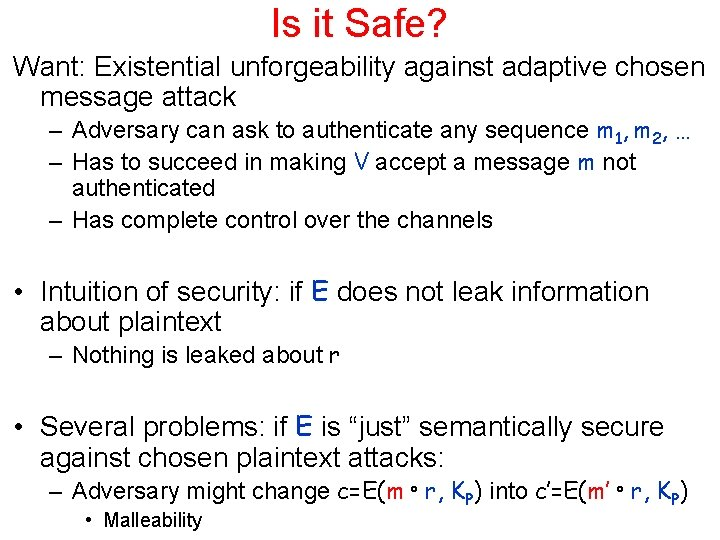Is it Safe? Want: Existential unforgeability against adaptive chosen message attack – Adversary can ask to authenticate any sequence m 1, m 2, … – Has to succeed in making V accept a message m not authenticated – Has complete control over the channels • Intuition of security: if E does not leak information about plaintext – Nothing is leaked about r • Several problems: if E is “just” semantically secure against chosen plaintext attacks: – Adversary might change c=E(m ° r, KP) into c’=E(m’ ° r, KP) • Malleability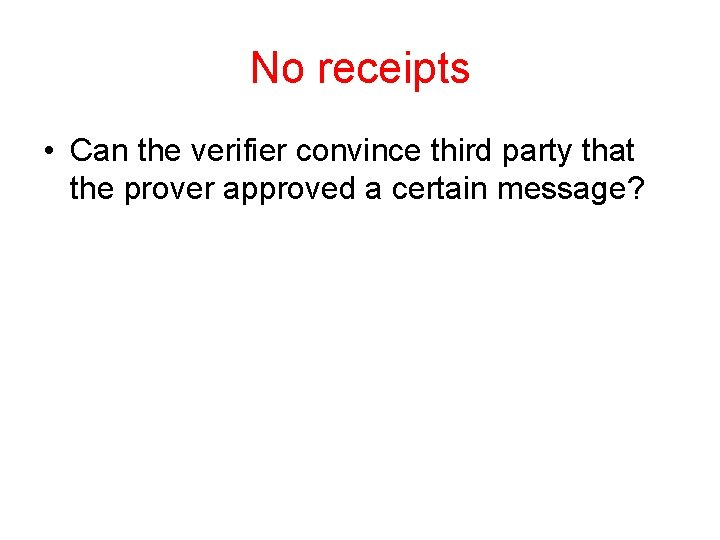No receipts • Can the verifier convince third party that the prover approved a certain message?Authentication and Non. Repudiation • Key idea of modern cryptography [Diffie. Hellman]: can make authentication (signatures) transferable to third party - Non-repudiation. – Essential to contract signing, e-commerce… • Digital Signatures: last 25 years major effort in – Research • Notions of security • Computationally efficient constructions – Technology, Infrastructure (PKI), Commerce,Is non-repudiation always desirable? Not necessarily so: • Privacy of conversation, no (verifiable) record. – Do you want everything you ever said to be held against you? • If Bob pays for the authentication, shouldn't be able to transfer it for free • Perhaps can gain efficiency Alternative: (Plausible) Deniability If the recipient (or any recipient) could have generated the conversation himself or an indistinguishable one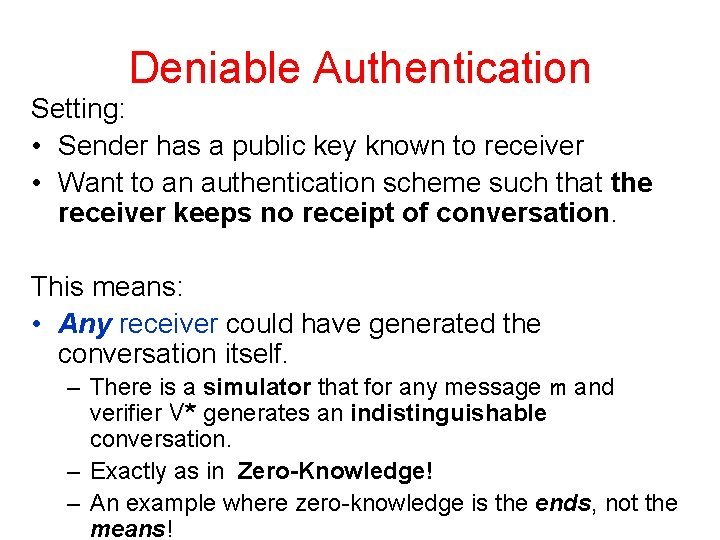Deniable Authentication Setting: • Sender has a public key known to receiver • Want to an authentication scheme such that the receiver keeps no receipt of conversation. This means: • Any receiver could have generated the conversation itself. – There is a simulator that for any message m and verifier V* generates an indistinguishable conversation. – Exactly as in Zero-Knowledge! – An example where zero-knowledge is the ends, not the means!Ring Signatures and Authentication Can we keep the sender anonymous? Idea: prove that the signer is a member of an ad hoc set – Other members do not cooperate – Use their `regular’ public-keys • Encryption – Should be indistinguishable which member of the set is actually doing the authentication Bob Alice? EveA Public Key Authentication Protocol P has a public key PK of an encryption scheme E. To authenticate a message m: • V P : Choose r R {0, 1}n and random bits 2{0, 1}* Send Y=E(PK, m°r, ) • P V : Verify that prefix of plaintext is indeed m. If yes - send r. V accepts iff the received r’=r Is it Unforgeable? Is it DeniableSecurity of the scheme Unforgeability: depends on the strength of E • Sensitive to malleability: – if given E(PK, m°r, ) can generate E(PK, m’°r’, ’) where m’ is related to m and r’ is related to x then can forge. • The protocol allows a chosen ciphertext attack on E. – Even of the post-processing kind! • Can prove that any strategy for existential forgery can be translated into a CCA strategy on E • Works even against concurrent executions. We saw an encryption scheme satisfying the desired requirements Deniability: does V retain a receipt? ? – It does not retain one for an honest V – Need to prove knowledge of rSimulator for honest receiver Choose r R {0, 1}n. Output: h. Y=E(PK, m°r, ), x, i Has exactly the same distribution as a real conversation when the verifier is following the protocol Statistical indistinguishability Verifier might cheat by checking whether certain ciphertext have as a prefix m No known concrete way of doing harm this way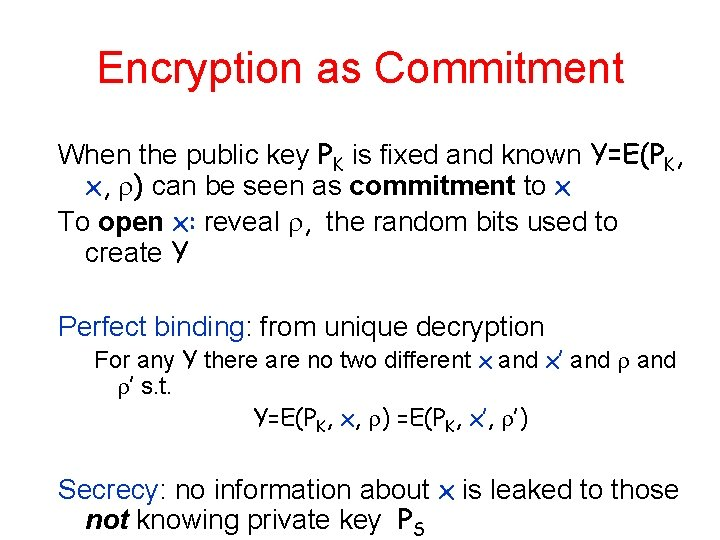Encryption as Commitment When the public key PK is fixed and known Y=E(PK, x, ) can be seen as commitment to x To open x: reveal , the random bits used to create Y Perfect binding: from unique decryption For any Y there are no two different x and x’ and ’ s. t. Y=E(PK, x, ) =E(PK, x’, ’) Secrecy: no information about x is leaked to those not knowing private key PS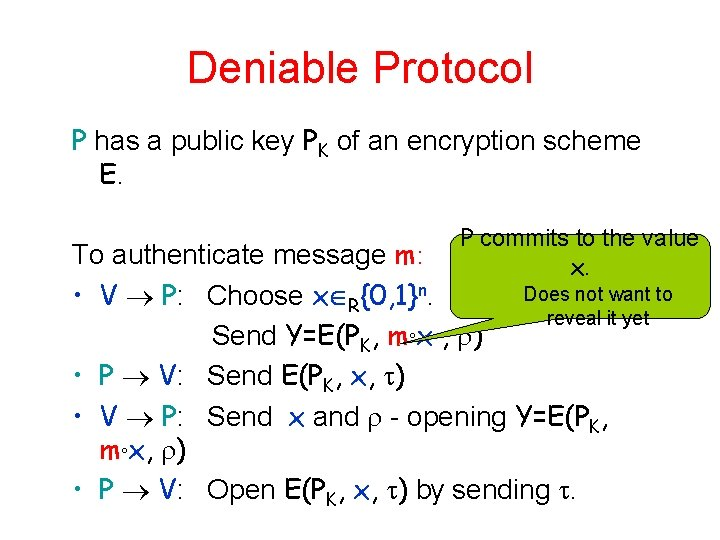Deniable Protocol P has a public key PK of an encryption scheme E. P commits to the value x. To authenticate message m: Does not want to • V P: Choose x R{0, 1}n. reveal it yet Send Y=E(PK, m°x , ) • P V: Send E(PK, x, ) • V P: Send x and - opening Y=E(PK, m°x, ) • P V: Open E(PK, x, ) by sending .Security of the scheme Unforgeability: as before - depends on the strength of E can simulate previous scheme (with access to D(PK , . )) Important property: E(PK, x, ) is a non-malleable commitment (wrt the encryption) to x. Deniability: can run simulator: • Extract x by running with E(PK, garbage, ) and rewinding • Expected polynomial time • Need the semantic security of E - it acts as a commitment scheme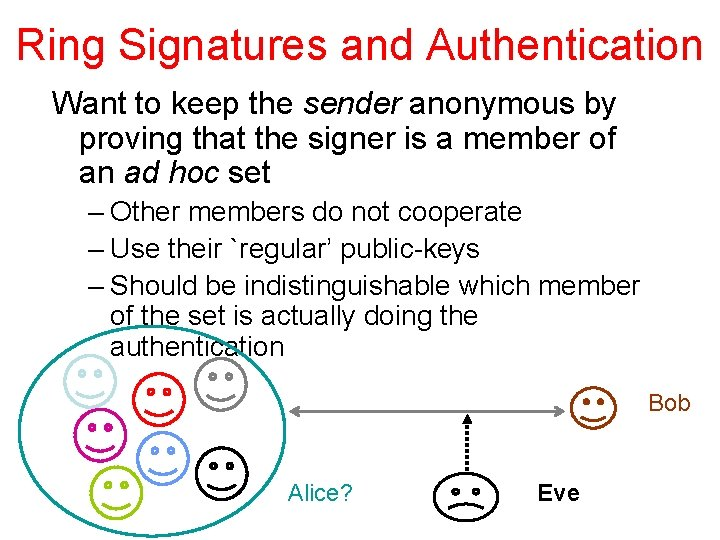Ring Signatures and Authentication Want to keep the sender anonymous by proving that the signer is a member of an ad hoc set – Other members do not cooperate – Use their `regular’ public-keys – Should be indistinguishable which member of the set is actually doing the authentication Bob Alice? Eve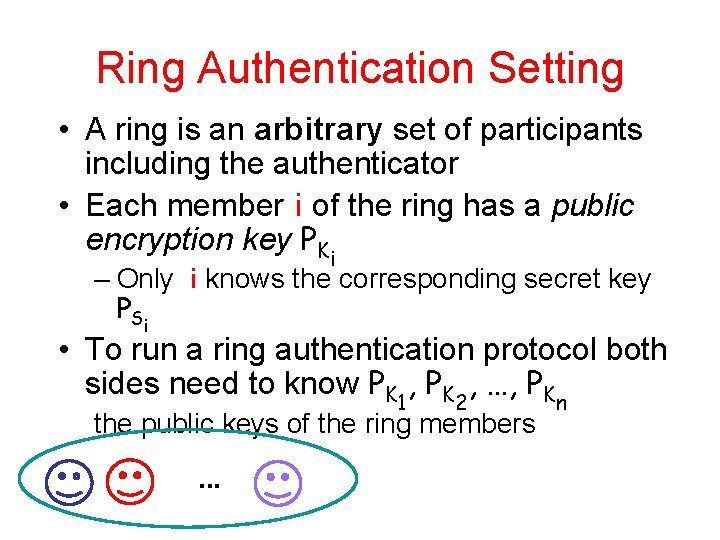Ring Authentication Setting • A ring is an arbitrary set of participants including the authenticator • Each member i of the ring has a public encryption key PKi – Only i knows the corresponding secret key P Si • To run a ring authentication protocol both sides need to know PK 1, PK 2, …, PKn the public keys of the ring members. . .An almost Good Ring Authentication Protocol Ring has public keys PK 1, PK 2, …, PKn of encryption scheme E To authenticate message m with jth decryption key PSj: V P: Choose x {0, 1}n. Send E(PK 1, m°x, r 1), E(PK 2, m°x, r 2), …, E(PKn, m°x, rn) P V: Decrypt E(PKj, m°x, rj), using PSj and Send E(PK 1, x, 1), E(PK 2, x, 2), …, E(PKn, x, n) V P: open all the E(PKi, m°x, ri) by Send x and r 1, r 2 , … rn P V: Verify consistency and open all E(PKi, x, ti) by Send t 1, 2 , … n Problem: what if not all suffixes (x‘s) are equal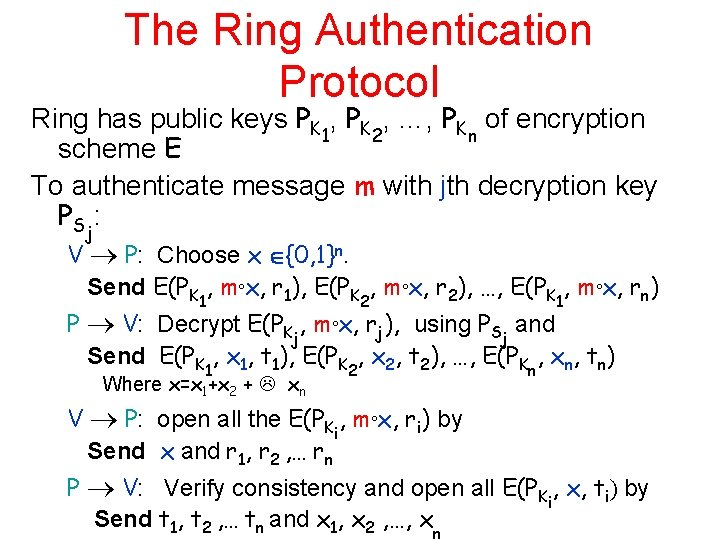The Ring Authentication Protocol Ring has public keys PK 1, PK 2, …, PKn of encryption scheme E To authenticate message m with jth decryption key PS : j V P: Choose x {0, 1}n. Send E(PK 1, m°x, r 1), E(PK 2, m°x, r 2), …, E(PK 1, m°x, rn) P V: Decrypt E(PKj, m°x, rj), using PSj and Send E(PK 1, x 1, t 1), E(PK 2, x 2, t 2), …, E(PKn, xn, tn) Where x=x 1+x 2 + xn V P: open all the E(PKi, m°x, ri) by Send x and r 1, r 2 , … rn P V: Verify consistency and open all E(PKi, x, ti) by Send t 1, t 2 , … tn and x 1, x 2 , …, xnComplexity of the scheme Sender: single decryption, n encryptions and n encryption verifications Receiver: n encryptions and n encryption verifications Communication Complexity: O(n) public-key encryptionsSecurity of the scheme Unforgeability: as before (assuming all keys are well chosen) since E(PK 1, x 1, t 1), E(PK 2, x 2, t 2), …, E(PK 1, xn, tn) where x=x 1+x 2 + xn is a non-malleable commitment to x Source Hiding: which key was used (among well chosen keys) is – Computationally indistinguishable during protocol – Statistically indistinguishable after protocol • If ends successfully Deniability: Can run simulator `as before’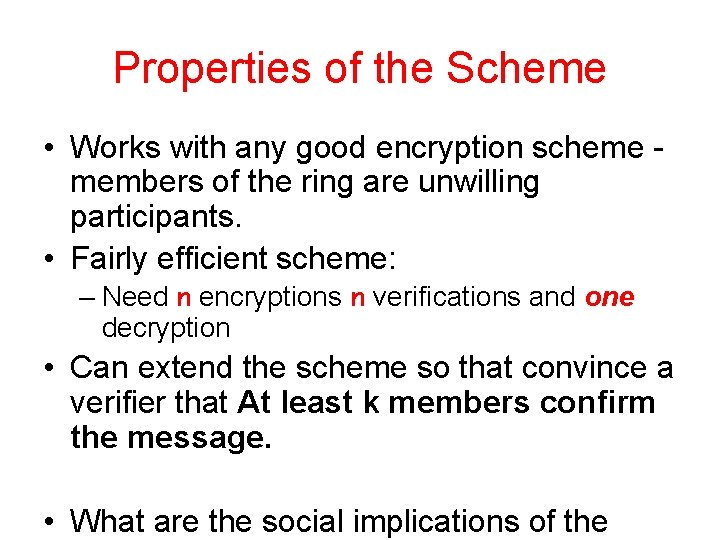Properties of the Scheme • Works with any good encryption scheme members of the ring are unwilling participants. • Fairly efficient scheme: – Need n encryptions n verifications and one decryption • Can extend the scheme so that convince a verifier that At least k members confirm the message. • What are the social implications of theSources • Dolev, Dwork and Naor: Non Malleable Cryptography, Siam J. computing 2000. also Siam Review 2003 • Cramer and Shoup: Design and analysis of practical public-key encryption schemes secure against adaptive chosen ciphertext attack (see www. shoup. net) • Lindell: A Simpler Construction of CCA 2 Secure Public-Key Encryption Under General Assumptions. In Eurocrypt 2003,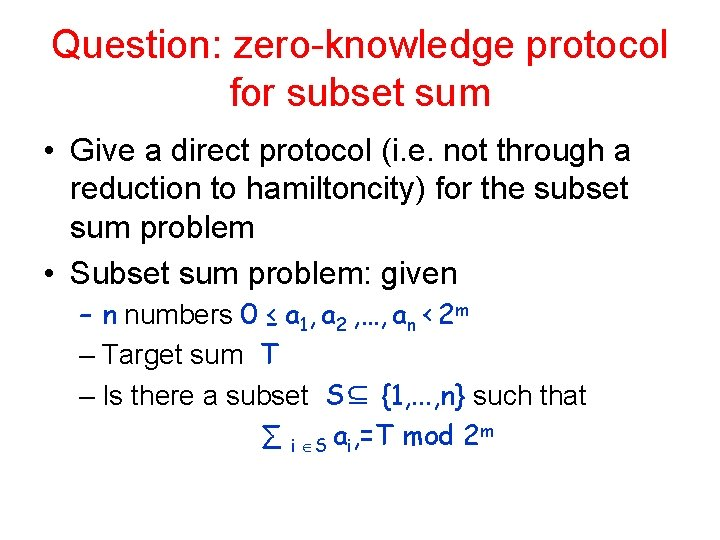Question: zero-knowledge protocol for subset sum • Give a direct protocol (i. e. not through a reduction to hamiltoncity) for the subset sum problem • Subset sum problem: given – n numbers 0 ≤ a 1, a 2 , …, an < 2 m – Target sum T – Is there a subset S⊆ {1, . . . , n} such that ∑ i S ai, =T mod 2 mQuestion: statistically hiding, computationally biding commitments from collision intractable hash functions • Goal: construct a commitment scheme where • the induced distribution of the transcript is (nearly independent of the string committed to • No PPT sender can with probability (1 -negligible) reveal two different strings following the commit phase. Protocol for committing to a bit b. Let H be a family of collision intractable hash functions Inner product Commit: over GF • Receiver: choose h 2 H and give to sender • Sender: choose random z and r. Send h(z), r and hz¢ri©b Reveal: publish z Prove that the protocol satisfies the above requirementsFind the error Let E be an public-key encryption scheme which is errorless. Let H be a family of collision intractable hash functions Commit: Sender chooses • Key for E(KP, KS) and h 2 H and sends h, KP, E(KP, x), h(x ◦ E(Kp, x)) Reveal: publish KS Assuming collision intractable hash functions exists Show that there exists a family H be a family of collision intractable hash functions such that the scheme is insecure for any E.